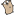## Go by Example 中文：变参函数

 可变参数函数。可以用任意数量的参数调用。例如，`fmt.Println` 是一个常见的变参函数。```package main ``` ```import "fmt" ``` 这个函数使用任意数目的 `int` 作为参数。 ```func sum(nums ...int) { fmt.Print(nums, " ") total := 0 for _, num := range nums { total += num } fmt.Println(total) } ``` ```func main() { ``` 变参函数使用常规的调用方式，除了参数比较特殊。 ``` sum(1, 2) sum(1, 2, 3) ``` 如果你的 slice 已经有了多个值，想把它们作为变参使用，你要这样调用 `func(slice...)`。 ``` nums := []int{1, 2, 3, 4} sum(nums...) } ```
 ```\$ go run variadic-functions.go [1 2] 3 [1 2 3] 6 [1 2 3 4] 10 ``` Go 函数的另一个关键的方面是闭包结构，这是接下来我们需要看看的。